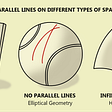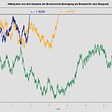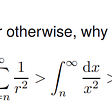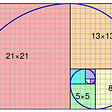# Part 4: Determinants

## How areas are changed

At this point, I have covered most of the topics that I want to go over in my presentation. This section will be a short, sweet overview of a fun topic that will be useful for a longer, more challenging article. We’ll be covering the determinant.

## Determinant Imagination

A determinant is simply how much a linear transformation changes the area of a given chunk compared to its original area formed by the basis vectors. Imagine for a moment the area of any square within the basis vectors. Now, imagine we apply a linear transformation that stretches i-hat by 5and j-hat by 2. This would increase the area of our original square by a factor of 10, so the determinant of that linear transformation, or its matrix, is 10. If we were to add another dimension (3D), the determinant would represent how much volume is scaled. It is also important to know that when the determinant is 0, that means that all of the area within the basis vectors is squeezed down into one point or line. This will help with eigenvectors and eigenvalues.

## Math Formula

For my introductory lesson, I will only be considering the simplest kinds of matrices: 2x2 ones. For these matrices, there is a nice formula that can quickly give us the determinant value. Note that the common notation for the determinant is “det” followed by parentheses around the matrix. View the formula below.

Now let’s try a simple example. Say w = 10, x = 2, y = 3, and z = 5. What would the determinant of this matrix be? Well, there’s no trick to this problem, as we simply just have to plug numbers into our formula. After doing so, we can see that the answer is 44.

Let’s do two more examples. First, let’s say w = 3, x = 9, y = 2, and z = 6. Going through the same process as before, we will calculate the determinant. In this case, we see that our answer is 0. As mentioned before, this means that our linear transformation squeezes area onto either a single point or a line (in most cases, it is a line).

Finally, let’s say w = 8, x = 7, y = 4, and z = 2. Again, we go through the same process to find the determinant. After crunching the numbers, we can see that we arrive at a negative number. Wait… What does this mean? It can be kind of strange to think of a negative area, but in a sense, that is what is going on. Notice how the basis vector i-hat is initially to the right of j-hat. When we apply a linear transformation that does not change this fact, the determinant is positive. However, if the linear transformation makes it so that i-hat ends up on the left of j-hat, then that means that the determinant will be negative. In other words, when we “invert” space, the determinant is negative. When I first learned this, I equated this negative determinant to that of an integral being negative. Even though I know that these two concepts can not be directly correlated, the relationship helped me make sense of when “area” can be thought of as negative. The work to the previous question can be found below.

## Final Thoughts

I hope this short article helped with the basics of the determinant. At this point in time, I am saving a few “extra topics” for my presentation, including eigenvectors and eigenvalues. Therefore, I needed to do a quick bit of learning on the determinant, as I will need it for when I teach eigenvectors. Overall, I had fun reading and solving practice problems. I know determinants will be useful for the rest of linear algebra, so I’m glad that I’ve gotten some decent exposure early on.

--

--

--

## More from Albert Ming

18 || High School Senior || Stats & Math

Love podcasts or audiobooks? Learn on the go with our new app.

## The Brownian Bridge Process## Gödel’s First Incompleteness Theorem## Vulcan Forge will melt faces due to insane yield potential.## Why You Should Learn Calculus## The Normal Distribution Simplified## My Linear Algebra Journey Begins Now## An Introduction to Probability and the World of Chance## Continuous Time Martingales for Quantitative Finance## Albert Ming

18 || High School Senior || Stats & Math

## Part 5: Revisiting Systems of Linear Equations## Try This Analysis Question!## Is math invented or discovered?## The fuss about “Necessary” and “Sufficient” in Mathematics